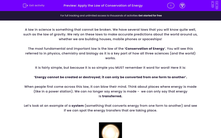# Carry Out Calculations Using Square Numbers

In this worksheet, students will add, subtract and divide square numbers.Key stage:  KS 3

Curriculum topic:   Number

Curriculum subtopic:   Understand Integer Powers/Real Roots

Popular topics:   Square Numbers worksheets

Difficulty level:#### Worksheet Overview

For this activity, it will be useful for you to know the square numbers off by heart.

12 = 1

22 = 4

32 = 9

42 = 16

52 = 25

62 = 36

72 = 49

82 = 64

92 = 81

102 = 100

112 = 121

122 = 144

It's also useful to know that 152 = 225

Example

Work out the following mentally:

52 + 10 = 25 + 10 = 35

The order in which we carry out calculations matters, so it will help if you can remember the order of BIDMAS:

Brackets

Indices or Powers

Division

Multiplication

Subtraction

We will be doing a variety of calculations involving square numbers, so time to get your brain in gear!### What is EdPlace?

We're your National Curriculum aligned online education content provider helping each child succeed in English, maths and science from year 1 to GCSE. With an EdPlace account you’ll be able to track and measure progress, helping each child achieve their best. We build confidence and attainment by personalising each child’s learning at a level that suits them.

Get started